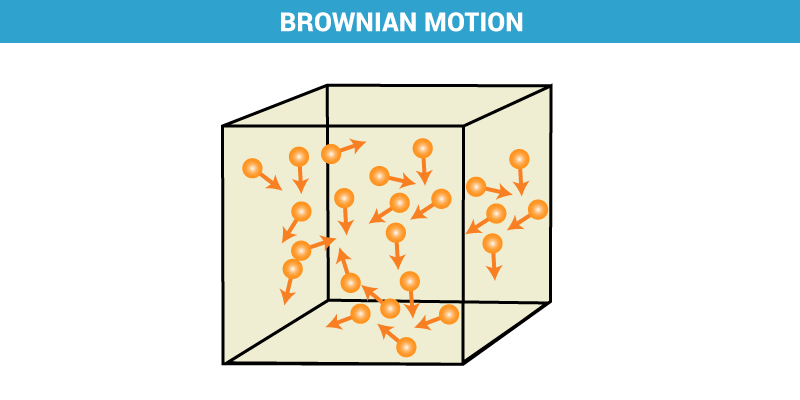# Brownian Motion

## What is the Brownian Motion?

The Brownian motion is named after a British Botanist Robert Brown who first observed it. It is also known as Brownian movement.

Brownian Motion can be defined as:

The random motion of particles suspended in a fluid resulting from their collision with the fast-moving molecules in the fluid.

In simple words, it’s just a random motion shown by small particles suspended in a fluid (liquid or gas) as shown below:Brownian Motion

As evident from the above-shown figure, a particle collides with other particles and thus changes its path. This interaction between particles leads to their random motion which is also commonly known as zig-zag motion. Brownian motion is a transport phenomenon which is, it transfers or exchanges mass, momentum or energy between the particles.

## What causes Brownian Motion?

We know that the unbalanced interactions between the particles cause the Brownian motion. Now let us try to understand the factors that affect this motion.

• Size of particles: Smaller the size of particles, faster is the motion. The reason behind this behaviour is that momentum transfer is inversely proportional to mass. So lighter the particle, more speed it will get after a collision.
• Viscosity: Viscosity is fluid’s resistance to flow. For water, it is less, but for toothpaste it is high. The viscosity is also inversely proportional to the speed of Brownian motion. So lesser the viscosity, faster the motion.

## Effects of Brownian Motion

1. The Brownian motion acts as a stirring system and thus doesn’t allow the particles to settle down. This leads to stability of colloidal solutions.
2. This also helps in distinguishing between the true solution and colloidal solutions.## Why are we studying Brownian Motion?

• The Brownian motion observed under the microscope was first real proof of the existence of atoms and molecules.
• The kinetic theory of gases which explains the pressure, temperature and volume of gases are based on Brownian motion model of particle.
• The mathematical model of Brownian motion has numerous real-world applications. For instance, stock market fluctuations are often understood by this model.

The Brownian motion describes randomness and chaos. This is one of the simplest models of randomness. Hence have many applications in real-world as most of the things on the miniature level are random and have some entropy associated with them. Thus the study of Brownian motion is critical in understanding the real world phenomenon.

## Einstein’s Theory:

Albert Einstein in 1905 gave his theory of Brownian motion after concluding another theory for the same. looking at the idea that the temperature of any substance is directly proportional to its average kinetic energy which these molecules move, it is obvious that this motion is imparted to a larger substance which could be seen under a microscope.

Einstein concluded a diffusion equation from his observation where the diffusion coefficient is similar to the mean squared displacement of the Brownian’s particle. This leads him to determine the size of atoms and the number of atoms present in a particle.